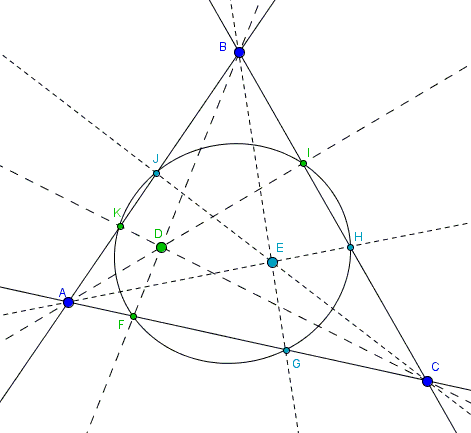Two Triples of Concurrent Cevians and a Conic

12 July 2013, Created with GeoGebra

Problem

In a triangle there are two pairs of concurrent cevians that - between them - have six feet on the side lines of a triangle.Prove that there is a conic through all six points.

Hint

This is a generalization of a conic tangent to the side lines at the feet of concurrent cevians that was established with homogeneous coordinates. It must be possible to adapt that method for the current problem.

Solution

There are two basic references at the site that introduce the tools I'll use below:

Let there be two points in the plane of a given triangle with coordinates $\alpha_{i}:\beta_{i}:\gamma_{i},$ $i=1,2.$

A conic in homogeneous coordinates $x:y:z$ has an equation of the form:

$f(x:y:z) = ax^{2}+by^{2}+cz^{2}+dyz+exz+fxy=0$

The task is to determine coefficients $a,b,c,d,e,f\space$ for which the conic passes through the six points $0:\beta_{i}:\gamma_{i},$ $\alpha_{i}:0:\gamma_{i},$ $\alpha_{i}:\beta_{i}:0,$ $i=1,2.$ We have six parameters $\alpha_{i},\beta_{i},\gamma_{i},$ $i=1,2$ and six coefficients to determine, $a,b,c,d,e,f.$ The possible problem lies in that only five coefficients are independent. So, unless, there is some relation between the six equations that we are going to consider, the problem will be unsolvable. But it is.

For the side $BC$ we obtain two equations

$f(0:\beta_{i} :\gamma_{i}) = b\beta_{i}^{2}+c\gamma_{i}^{2}+d\beta_{i}\gamma_{i}=0,$ $i=1,2,$

from which $\displaystyle d=-\frac{b\beta_{i}^{2}+c\gamma_{i}^{2}}{\beta_{i}\gamma_{i}}.$ For there to be consistency, we must request that

$\displaystyle \frac{b\beta_{1}^{2}+c\gamma_{1}^{2}}{\beta_{1}\gamma_{1}}=\frac{b\beta_{2}^{2}+c\gamma_{2}^{2}}{\beta_{2}\gamma_{2}}$

This is satisfied when $b:c=\gamma_{1}\gamma_{2}:\beta_{1}\beta_{2}.$ Indeed, from the above

$\displaystyle b\frac{\beta_{1}}{\gamma_{1}}+c\frac{\gamma_{1}}{\beta_{1}}=b\frac{\beta_{2}}{\gamma_{2}}+c\frac{\gamma_{2}}{\beta_{2}}.$

And, therefore,

$\displaystyle b(\frac{\beta_{1}}{\gamma_{1}}-\frac{\beta_{2}}{\gamma_{2}})=c(\frac{\gamma_{2}}{\beta_{2}}-\frac{\gamma_{1}}{\beta_{1}}).$

Finally, we arrive at

$\displaystyle b\frac{\beta_{1}\gamma_{2}-\beta_{2}\gamma_{1}}{\gamma_{1}\gamma_{2}}=c\frac{\beta_{1}\gamma_{2}-\beta_{2}\gamma_{1}}{\beta_{1}\beta_{2}}.$

Similarly, we proceed with the points on the other two sides of the triangle:

$c:a=\alpha_{1}\alpha_{2}:\gamma_{1}\gamma_{2},$ and
$a:b=\beta_{1}\beta_{2}:\alpha_{1}\alpha_{2}$

Obviously, the three proportions are not independent. All together can be written as

$a:b:c=\beta_{1}\beta_{2}\gamma_{1}\gamma_{2}:\gamma_{1}\gamma_{2}\alpha_{1}\alpha_{2}:\alpha_{1}\alpha_{2}\beta_{1}\beta_{2}.$

When the points $\alpha_{i}:\beta_{i}:\gamma_{i},$ $i=1,2$ approach each other, so do their feet on the corresponding side lines. When the points coincide the conic becomes tangent to the latter, retrieving the earlier result.

The inverse of sorts of this result is naturally true:

Assume there is a conic through six points (two on a side of a triangle) and the cevians to three of the points are concurrent. Then the cevians to the remaining three are also concurrent.

Indeed, let say $AH,BG,CJ$ are concurrent; $AI, BF, CK$ are the remaining cevians that may be not. Draw the cevian $CK'$ concurrent with $AI$ and $BF.$ By what we just proved there is a unique conic through $H,G,J,I,F,K'.$ But there is already a conic through $H,G,J,I,F,K;$ it is also known that five distinct points define a unique conic. If $K\ne K'$ then a conic would cross a straight line $AB$ in three points, which is impossible. It follows that $K=K',$ making $AI, BF, CK$ concurrent.

This is a standard situation where a sufficient condition is automatically necessary.

Acknowledgment

I am very grateful to Hubert Shutrick for his continuing interest in the site and stimulating discussions.